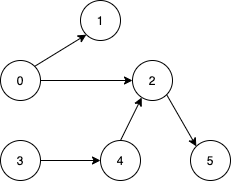# 1557. Minimum Number of Vertices to Reach All Nodes

Medium
Given a directed acyclic graph, with `n` vertices numbered from `0` to `n-1`, and an array `edges` where `edges[i] = [fromi, toi]` represents a directed edge from node `fromi` to node `toi`.
Find the smallest set of vertices from which all nodes in the graph are reachable. It's guaranteed that a unique solution exists.
Notice that you can return the vertices in any order.
Example 1:Input: n = 6, edges = [[0,1],[0,2],[2,5],[3,4],[4,2]]
Output: [0,3]
Explanation: It's not possible to reach all the nodes from a single vertex. From 0 we can reach [0,1,2,5]. From 3 we can reach [3,4,2,5]. So we output [0,3].
Example 2:Input: n = 5, edges = [[0,1],[2,1],[3,1],[1,4],[2,4]]
Output: [0,2,3]
Explanation: Notice that vertices 0, 3 and 2 are not reachable from any other node, so we must include them. Also any of these vertices can reach nodes 1 and 4.
Constraints:
• `2 <= n <= 10^5`
• `1 <= edges.length <= min(10^5, n * (n - 1) / 2)`
• `edges[i].length == 2`
• `0 <= fromi, toi < n`
• All pairs `(fromi, toi)` are distinct.

### 解題

Runtime: 166 ms, faster than 100%
Memory Usage: 16.1 MB, less than 96.97%
func findSmallestSetOfVertices(n int, edges [][]int) []int {
indegree := make([]int, n)
for _, edge := range edges {
indegree[edge]++
}
ans := make([]int, 0)
for i, n := range indegree {
if n == 0 {
ans = append(ans, i)
}
}
return ans
}